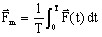# Pulsating quantities: power, force...

## Definition: reminder

A pulsating quantity is a periodic quantity with non-null mean value.

Two examples:

• The instantaneous electric power absorbed or supplied by a dipole is the sum of two components:
• a time independent component: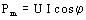• a periodic component (of double frequency):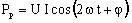• The instantaneous force exerted on the blade of a contactor is the sum of two components:
• a time independent component: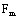• a periodic component (of double frequency):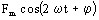## Post-processing of the scalar pulsating quantities (power…)

For the scalar quantity as the power, the main interest is on the constant component (mean value). The pulsating term is of little interest.

The post-processing can be carried out in different ways:

• instantaneous post-processing (at a given ω t moment):
• value of the quantity P(t)
• post-processing using the time integration :
• mean value
•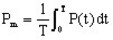The instantaneous power P(t) is not available in Flux at the 2D and 3D postprocessors level.

## Post-processing of the vector pulsating quantities (force…)

For a vector quantity as the force, the two components, the constant (mean value) and the pulsating one, are interesting.

The post-processing can be carried out in different ways:

• instantaneous post-processing (at a given moment ωt):
• value of the vector quantity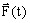• post-processing using the time integration :
• mean value
•